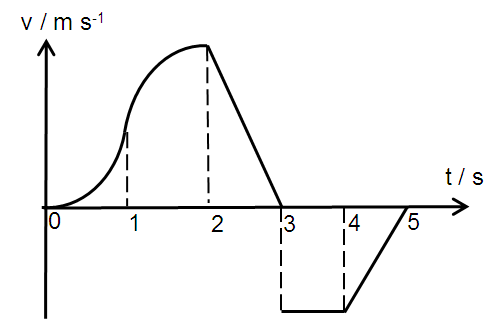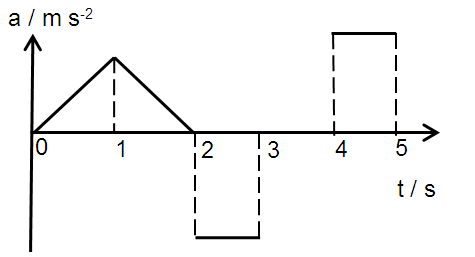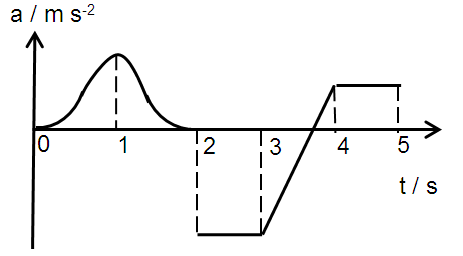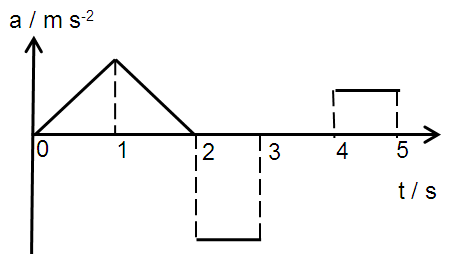# Acceleration-time graphs in 1D kinematics

A body which is initially at rest experiences a change in velocity and travels in a straight horizontal line as shown below (graph drawn to scale, take positive v as rightwards motion).Which of the following shows the correct graph for the acceleration of the body over the same time period, and the sign of the displacement of the body after 5 s?

Acceleration Graph AAcceleration Graph BAcceleration Graph C×